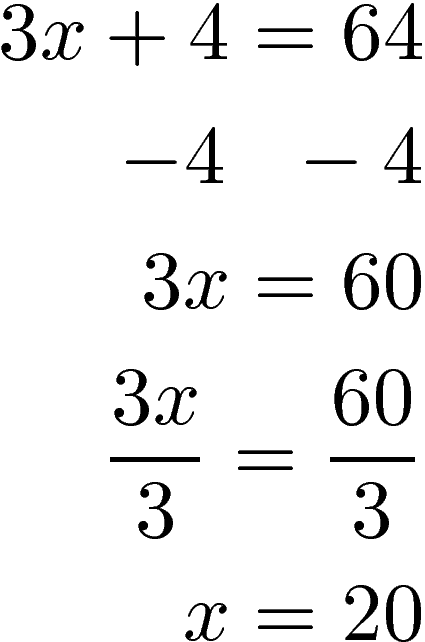# Linear Equations

In this post, we will look at several types of equations that you would encounter when learning algebra. Algebra is a foundational subject to know when conducting most quantitative research.Equations

An equation is a statement that balances two expressions. Often equations include a variable or an unknown value. By solving for the unknown value you are able to balance the equation.

There are many different types of equations such as

(1) Linear equation(3) Polynomial equation

See number 1 or 2. The rule for polynomial equation is that the exponent must be positive
(4) Trigonometric equation(6) Exponential equationThis post will focus on linear equations.

Linear Equation

A linear equation is an equation that if it is graph will render a straight line. It is common to have to solve for the variable in a linear equation by isolating as in the example below.There are also several terms related to equations and the include the following

• Conditional equation: An equation that is true for only one value of the variable. The example above is a conditional equation.
• Identity: An equation that is true for any value of a variable. Below is an exampleAny value of x will work with an identity equation.

• A contradiction is an equation that is false for all values. Below is an exampleNo value of x will work with the equation above.

Conclusion

This post provided an overview of the types of equations commonly encountered in algebra.